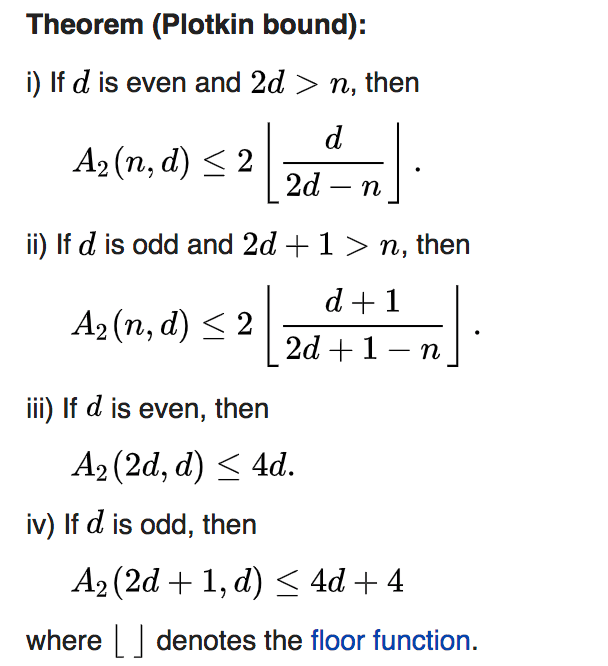# A problem on binary orthogonal matrix

Contents

## Problem$H$ is a binary square matrix whose elements are chosen from the set$\{1, -1\}$. That is$H \in \{1, -1\}^{(n \times n)}$. And$H$ is an orthogonal matrix,$HH^T=nI$.

Theorem: prove that any sub matrix$S$ of$H$, with size$a \times b$, if this sub matrix$S$ contains only$1$(no$-1$ in it at all), then$ab \le n$.

## Proof

Consider any sub matrix of$S \in \{1\}^{a \times b}$ of$H$. Let’s focus on the$a$ row vectors of$H$. And all these$a$ vectors’ column$i\sim i+b-1$ contains only 1.

Let’s eval the Hamming Distance between each pair of these row vectors. Each row vector consists of two parts:

•$i\sim i+b-1$(this part is the same for all these row vectors).
• the remaining columns(this is the part that will cause Hamming Distance)

Taking any two row vectors, let

•$x$ be the number of the columns in the second part that the elements in the two vectors have the same sign
•$y$ be the number of the columns in the second part that the elements in the two vectors have the different sign

We have:

•$x+y=n-a$, (the length of the second part)
•$a + x - y = 0$, (the two vectors are orthogonal)

So$y=\frac{n}{2}$.

Now let’s only consider the vectors contain only the second-part columns. These vectors’ length is$n-b$. There are$a$ such vectors. And the pair-wise Hamming Distance is$\frac{n}{2}$.

Let’s model this a coding problem and find the relation among codewords number, codewords length and Hamming Distance.

There is a famous theorem Plotkin Bound for the problem:Pay attention to the symbols and do not mix them up:

•$n$ in the above picture means the codeword’s length,$n-b$ inour case
•$d$ in the above picture means the minimal Hamming Distance,$\frac{n}{2}$ in our case
•$A$ in the above picture means the the codewords number,$a$ in our case

If$d$ is even, then$2d=n>(n-b)$, so we can use i) of the theorem. That is,$a \le \frac{n}{b}$, this is just what we want to prove.

If$d$ is odd, then$2d+1=n+1>(n-b)$, so we can use ii) of the theorem. That is$a \le \frac{n+2}{b+1} \implies ab \le n + 2 - a$:

• if$a = 1$, it is obvious that$ab \le n$
• if$a \ge 2$,$ab \le n + 2 - a \le n$

So we prove that$ab \le n$.

## Plotkin Bound

Please refer wikipedia link for more details: Plotkin BoundIt is quite easy to prove and understand. The core idea is to represent the summation of all pairs’ Hamming Distance in two different ways.

This site uses Akismet to reduce spam. Learn how your comment data is processed.